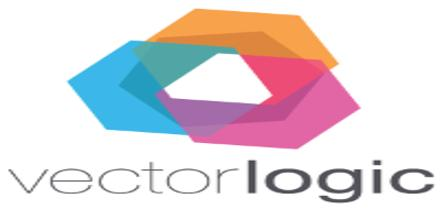Mathematic

# Vector LogicThis article talks about Vector Logic, which is a matrix–vector representation of the logical calculus inspired in neural network models. It is a direct translation into a matrix-vector formalism of the classical Boolean polynomials. This kind of formalism has been applied to develop a fuzzy logic in terms of complex numbers. It requires independent affirmative evidence for each assertion in a proposition, and does not make the assumption for binary complementation. Vector Logic also can be extended to include many truth values since large dimensional vector spaces allow to create many orthogonal truth values and the corresponding logical matrices.